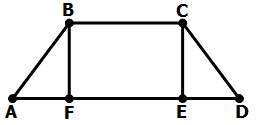Click text to edit
Add a note to this section

Passage Tools
SHOW HIDE
• Calculator
• FlagIn trapezoid ABCD, BC = EF = 6, AF = DE = 3, and

AB = CD = 5. If the area of a trapezoid is given by

then which of the following is the area of trapezoid ABCD?

Choose the option that best answers the question.
##### Title

Pythagorean Trapezoid Area

Correct

Medium